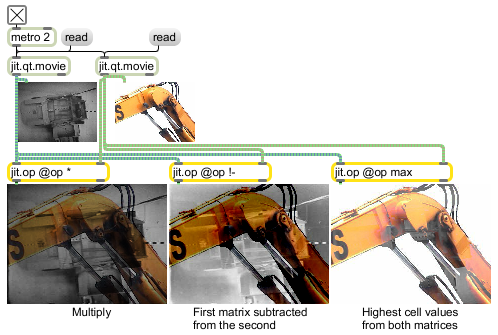# jit.op

Apply binary or unary operators

## Description

The jit.op object applies either a binary operator to two input matrices, or a unary operator to the left input matrix. A different operator may be specified for each plane, or a scalar may be specified via the val attribute as an alternate to using a second matrix.

## Matrix Operator

matrix inputs:2, matrix outputs:1
 Name IOProc Planelink Typelink Dimlink Plane Dim Type in2 resamp 1 1 1 1 1 char long float32 float64 out n/a 1 1 1 1 1 char long float32 float64

## Messages

 int Sets a value to int. float Sets a value to float. list Sets value to list.

## Attributes

Name Type g/s Description
val atom The value to use as right hand operand instead of a matrix (default = 0) If one value is specified it applies to all planes. If multiple values are specified they are applied on a plane by plane basis. IMPORTANT : If the incoming matrix data has typechar, then integers in the range 0-255 are mapped to the floating point data range 0.- .996 (A value of 1. (char 255) is not really 1. That value is not representable in 8-bit fixed point, since it would be 256. Thus, floating point values greater than 1. become 1.0 - 1/256.0, or 0.996).
op symbol The operator to be used (default = pass) If one operator is specified, it is applied to all planes. If multiple operators are specified, they are applied on a plane by plane basis.

Arithmetic Operators:
pass = pass left input, no operator
* = multiplication (also mult)
/ = division (also div)
- = subtraction (also sub)
-m = subtraction modulo (char only) (also subm)
% = modulo (also mod)
min = minimum
max = maximum
abs = absolute value (unary)
avg = average
absdiff = absolute value of difference
fold = folding/mirrored modulo (float only)
wrap = wrapping/positive modulo (float only)
!pass = pass right input, no operator
!/ = right input divided by left input (flipped)
!- = right input minus left input (flipped)
!% = right input modulo left input (flipped)
ignore = leave previous output value

Trigonometric Operators: (float32/float64 only, unary except atan2)
sin = sine
cos = cosine
tan = tangent
asin = arcsine
acos = arccosine
atan = arctangent
atan2 = arctangent (binary)
sinh = hyperbolic sine
cosh = hyperbolic cosine
tanh = hyperbolic tangent
asinh = hyperbolic arcsine
acosh = hyperbolic arccosine
atanh = hyperbolic arctangent

Bitwise Operators: (long/char only)
= bitwise and
| = bitwise or
^ = bitwise xor
~ = bitwise compliment (unary)
= right shift
= left shift

Logical operators
= logical and
|| = logical or
! = logical not (unary)
= greater than
= less than
= = greater than or equal to
= = less than or equal to
== = equal
!= = not equal
p = greater than (pass)
p = less than (pass)
=p = greater than or equal to (pass)
=p = less than or equal to (pass)
==p = equal (pass)
!=p not equal (pass)

Exponential/Logarithmic/Other: (float32/float64 only, unary except hypot and pow)
exp = e to the x
exp2 = 2 to the x
ln = log base e
log2 = log base 2
log10 = log base 10
hypot = hypotenuse (binary)
pow = x to the y (binary)
sqrt = square root
ceil = integer ceiling
floor = integer floor
round = round to nearest integer
trunc = truncate to integer

## Examples